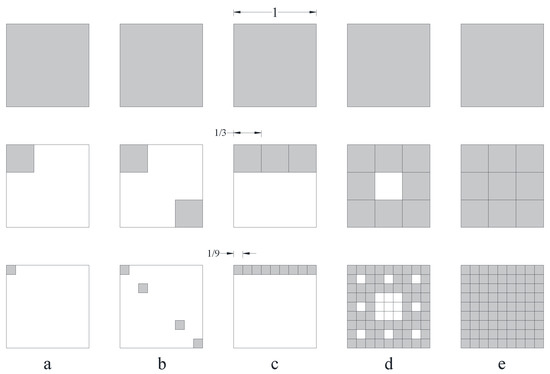Next Article in Journal
Moment Bound of Solution to a Class of Conformable Time-Fractional Stochastic Equation
Previous Article in Journal
An Application of the Sonine–Letnikov Fractional Derivative for the Radial Schrödinger Equation
Open AccessArticle

# Determination of the Fractal Dimension of the Fracture Network System Using Image Processing Technique

byRouhollah Basirat,Kamran Goshtasbi *andMorteza Ahmadi
Rock Mechanics Engineering Division, Faculty of Engineering, Tarbiat Modares University, 14115-111 Tehran, Iran
*
Author to whom correspondence should be addressed.
Fractal Fract 2019, 3(2), 17; https://doi.org/10.3390/fractalfract3020017
Received: 12 March 2019 / Revised: 3 April 2019 / Accepted: 4 April 2019 / Published: 8 April 2019
Fractal dimension (FD) is a critical parameter in the characterization of a rock fracture network system. This parameter represents the distribution pattern of fractures in rock media. Moreover, it can be used for the modeling of fracture networks when the spatial distribution of fractures is described by the distribution of power law. The main objective of this research is to propose an automatic method to determine the rock mass FD in MATLAB using digital image processing techniques. This method not only accelerates analysis and reduces human error, but also eliminates the access limitation to a rock face. In the proposed method, the intensity of image brightness is corrected using the histogram equalization process and applying smoothing filters to the image followed by revealing the edges using the Canny edge detection algorithm. In the next step, FD is calculated in the program using the box-counting method, which is applied randomly to the pixels detected as fractures. This algorithm was implemented in different geological images to calculate their FDs. The FD of the images was determined using a simple Canny edge detection algorithm, a manual calculation method, and an indirect approach based on spectral decay rate. The results showed that the proposed method is a reliable and fast approach for calculating FD in fractured geological media. View Full-Text
Keywords:
Show FiguresFigure 1

MDPI and ACS Style

Basirat, R.; Goshtasbi, K.; Ahmadi, M. Determination of the Fractal Dimension of the Fracture Network System Using Image Processing Technique. Fractal Fract 2019, 3, 17.